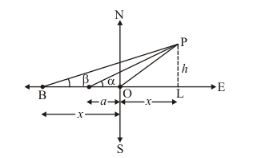# A tree standing on a horizontal plane is leaning towards east.

Question:

A tree standing on a horizontal plane is leaning towards east. At two points situated at distances a and b exactly due west on it,  the angles of elevation of the top are respectively α and β. Prove that the height of the top from the ground is

$\frac{(b-a) \tan \alpha \tan \beta}{\tan \alpha-\tan \beta}$

Solution:

Let OP be the tree and AB be the two points such OA = a and OB = b and angle of elevation to the tops are α and β respectively. Let OL = x and PL = h

We have to prove the following

$h=\frac{(b-a) \tan \alpha \tan \beta}{(\tan \alpha-\tan \beta)}$

The corresponding figure is as followsIn $\triangle A L P$

$\Rightarrow \quad \tan \alpha=\frac{P L}{O A+O L}$

$\Rightarrow \quad \tan \alpha=\frac{h}{a+x}$

$\Rightarrow \quad \frac{1}{\cot \alpha}=\frac{h}{a+x}$

$\Rightarrow \quad h \cot \alpha=a+x \ldots \ldots$ (1)

Again in$\Rightarrow \quad \tan \beta=\frac{P L}{O B+O L}$

$\Rightarrow \quad \tan \beta=\frac{h}{b+x}$

$\Rightarrow \quad \frac{1}{\cot \beta}=\frac{h}{b+x}$

$\Rightarrow \quad h \cot \beta=b+x \ldots \ldots$ (2)

Subtracting equation (1) from (2) we get

$\Rightarrow h \cot \beta-h \cot \alpha=b-a$

$\Rightarrow h(\cot \beta-\cot \alpha)=b-a$

$\Rightarrow \quad h=\frac{b-a}{\cot \beta-\cot \alpha}$

$h=\frac{(b-a) \tan \alpha \tan \beta}{(\tan \alpha-\tan \beta)}$

Hence height of the top from ground is $h=\frac{(b-a) \tan \alpha \tan \beta}{(\tan \alpha-\tan \beta)}$